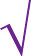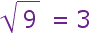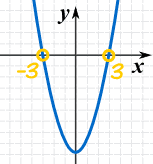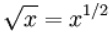# 代数里的平方与平方根

## 平方

### 例子：3 的平方是多少?

 3 的平方 == 3 × 3 = 9

"平方" 通常是写成一个小小的 2：（小的 2 代表数在乘法里出现了 2 次）

## 平方根3 的平方是 9，所以 9 的平方根是 3

x 的平方根是 平方为 x 的数

r2 = x
r 是平方根

## 平方根符号这是 "平方根" 的符号，它像个对勾（对号），几百年前本来是一点和向上一提。 它的名字是根号，看上去好像很了不起！## 负数

### 例子：负5的平方是多少？

• 算5的平方，然后取其负数？
• 还是 (−5) 的平方？

• 算5的平方，然后取负数： −(5×5) = −25
• (−5) 的平方： (−5)×(−5) = +25

### 例子（更正） (−5)2 是多少？

(−5) × (−5) = 25

（因为 负负得正)r2 = x
r 是平方根

(−5)2 = 25
(+5)2 = 25

## 两个平方根

……25 的平方根 of 可以是 5 ，也可以是 −5

### 例子：解 w2 = a

w = √a   或   w = −√a

36 的平方根是 6 −6

36 = 6 （不是−6）

## 正负号

 ± 是一个特殊符号，它的意思是 "正 或 负"，
 所以： w = √a   或   w = −√a 也可以写成： w = ±√a

## 简而言之

 若： r2 = x 则： r = ±√x

## 这为什么重要？

### 例子：解 x2 − 9 = 0开始： x2 − 9 = 0 把 9 移到右边： x2 = 9 取平方： x = ±√9 答案： x = ±3

"±" 符号告诉我们 "−3" 也是个答案。

### 例子：求 x：(x − 3)2 = 16

 开始： (x − 3)2 = 16 取平方根： x − 3 = ±√16 计算 √16: x − 3 = ±4 把 3 移到右边： x = 3 ± 4 答案： x = 7 或 −1

## xy 的平方根

xy = √xy

xy 两者都一定要大于或等于 0

### 例子：√(100×4) 是多少？

 √(100×4) = √(100) × √(4) = 10 × 2 = 20

 √8√2 = √(8×2) = √16 = 4

### 例子：√(−8 × −2) 是多少？

 √(−8 × −2) = √(−8) × √(−2) = ？？？

√(−8 × −2) = √16 = +4

## 二分之一的指数x 一定要大于或等于 0